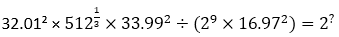SBI PO Quantitative Aptitude Quiz

Here, we are providing SBI PO Mains Study plan as there is left not enough time to deal in details. The questions asked in the quantitative aptitude section are calculative and very time-consuming. But once dealt with proper strategy, speed, and accuracy, this section can get you the maximum marks in the examination. Following is the Quantitative Aptitude quiz to help you practice with the best of latest pattern questions
For Queries related to Adda247 Ultimate for IBPS RRB 2019 Prelims, Click Here or mail us at ultimate@adda247.com

Directions (1-5): What approximate value should come in the place of the question mark (?) in the following questions? (You are not expected to calculate the exact value.)

Q1.121
441
1024
49
196

Q2.3
4
9
10
6

Q3. (14.99% of 4799.995) ÷ ? = (60.11% of 4.111)²
150
250
320
500
125

Q4.72
33
45
37
80

Q5.80
52
48
120
40

Directions (6-10): Table given below shows total number of applicants, who have applied for CDS exam in the five years. Percentage of applicants appeared in tier I exam, percentage of applicants qualified in tier I and tier II. Line graph shows percentage of applicants got selection in CDS exam. Read the data carefully and answer the questions:

Note – All applicants qualified in tier I appeared for tier II.

Q6. Number of applicants, who have got the final selection in the year 2017, is how many percent less than number of applicants, who have got the final selection in the year 2014?

Q7. Find the difference between number of applicants, who have got final selection in the years 2013 & 2016 together and number of applicants, who have got final selection in the year 2014 & 2015 together?
5
8
3
6
11

Q8. Find the ratio between applicants qualified in tier II but did not get final selection in the year 2017 to applicants qualified in tier I but did not qualify for tier II in the year 2014?
7 : 20
7 : 22
7 : 19
7 : 18
7 : 16

Q9. Total applicants appeared for tier I in the year 2017 is what percent more than total applicants appeared for tier II in the year 2015?

Q10. Find the total number of applicants, who did not qualify in tier I in the year 2014, 2016 & 2017 together?
10390
10590
10490
10290
10190

Q11. Total surface area of a cylinder mounted with a hemispherical bowl on one end is 2552 cm². If height of cylinder is 8 cm, then find the volume of the solid body? (cm3)

Q12. Train A running at the speed of 108 km/hr crosses a man, who running in the opposite direction at the speed of 12 km/hr in 7.2 sec. If speed of train A increased by 25% and it takes 48 seconds to cross another train B, which running at the speed of 90 km/hr in same direction. Find the length of train B?
280 meters
360 meters
180 meters
160 meters
220 meters

Q13. Vessel A contains (X + 24) l of mixture of milk and water in the ratio of 7 : 8, while vessel B contains (X + 54) l of same mixture of milk and water in the ratio of 3 : 2. If 37 ½ % of mixture from vessel A and 40% of mixture from vessel B taken out and mixed in vessel C, then the remaining mixture in vessel B is 15l more than that of in vessel A. find quantity of milk in vessel C?
49 litre
57 litre
55 litre
53 litre
51 litre

Directions (14 – 15): The following questions are accompanied by two statements (I) and (II). You have to determine which statements(s) is/are sufficient/necessary to answer the questions.

Q14. What is the population of the city A?
I. The ratio of the population of males and females in city A is 27 : 23 and the difference between their population is 100000.
II. The population of city A is 80% of that of city B. The difference between populations of city A and city B is 312500.
Statement (I) alone is sufficient to answer the question but statement (II) alone is not sufficient to answer the questions.
Statement (II) alone is sufficient to answer the question but statement (I) alone is not sufficient to answer the question.
Both the statements taken together are necessary to answer the questions, but neither of the statements alone is sufficient to answer the question.
Either statement (I) or statement (II) by itself is sufficient to answer the question.
Statements (I) and (II) taken together are not sufficient to answer the question.

Q15. What is the volume of the sphere?
I. Curved surface area of hemisphere is equal to the total surface area of the cylinder having radius and height in ratio 3 : 4.
II. When we cut sphere into two hemispheres then total surface area is equal to the area of a circle whose radius is 21 cm.
Statement (I) alone is sufficient to answer the question but statement (II) alone is not sufficient to answer the questions.
Statement (II) alone is sufficient to answer the question but statement (I) alone is not sufficient to answer the question.
Both the statements taken together are necessary to answer the questions, but neither of the statements alone is sufficient to answer the question.
Either statement (I) or statement (II) by itself is sufficient to answer the question.
Statements (I) and (II) taken together are not sufficient to answer the question.

You May also like to Read: